APP## 用户体验数据的多维度处理：PC A法及碎石图## 主成分分析法（PC A）设计逻辑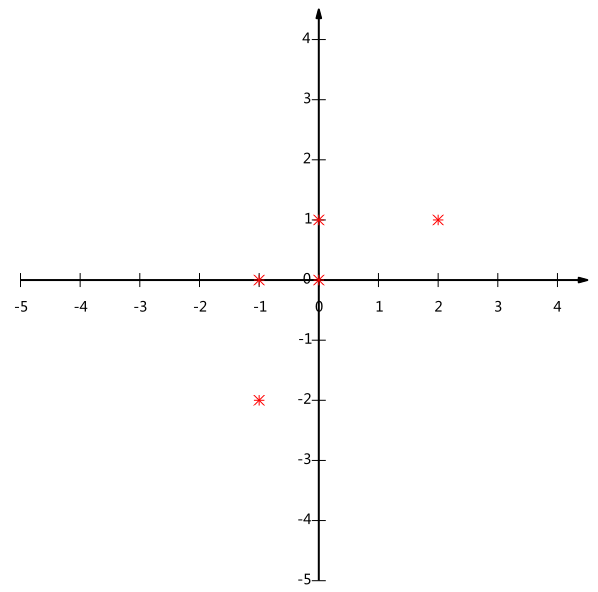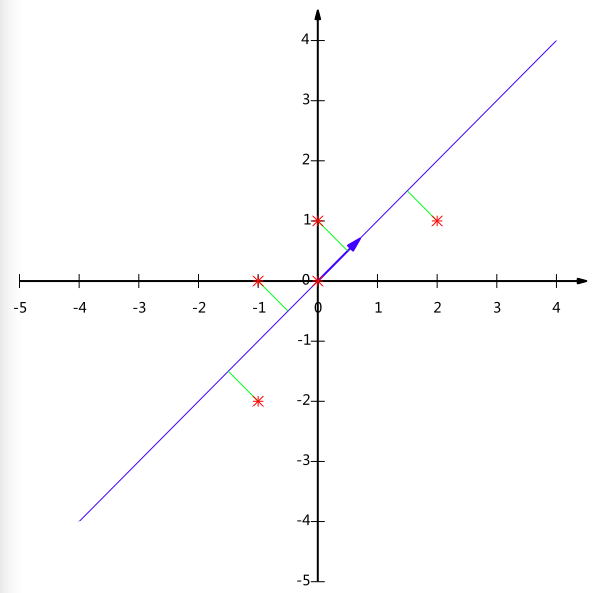## PC A实际案例分析

%data 为输入的数据集，行表示项目，项目之间是并行的例如产品1，产品2或者用户1，用户2，
%各列表示描述项目的维度比如，易用性，可用性，满意度等
data = shishen;

% PCA Template to data

% n 为测量的项目数量，p则表示描述的维度数量
[n,p] = size(data);

% PC A运算过程，这里就不再介绍，有兴趣可以参考《Exploratory Data Analysis with MATLAB》 。我这里将原数据集其降成一维。

datac = data – repmat(sum(data)/n,n,1);
covm = cov(datac);
[eigvec,eigval] = eig(covm);
eigval = diag(eigval);
eigval = flipud(eigval);
eigvec = eigvec(:,p:-1:1);
pervar = 100*cumsum(eigval)/sum(eigval);
g =zeros(1,p);
for k = 1:p
for i = k:p
g(k) = g(k) + 1/i;
end
end
g = g/p;
propvar = eigval/sum(eigval);
avgeig = mean(eigval);
ind = find(eigval > avgeig);
l=length(ind);

%P 控制转换成几个维度，若需要转换成X维，则p= eigvec(:,1:x);
P = eigvec(:,1:1);
%dataP 即是最后我们得到的降维之后的数据集
dataP = datac*P;

## 原数据说明### PC A处理结果及其意义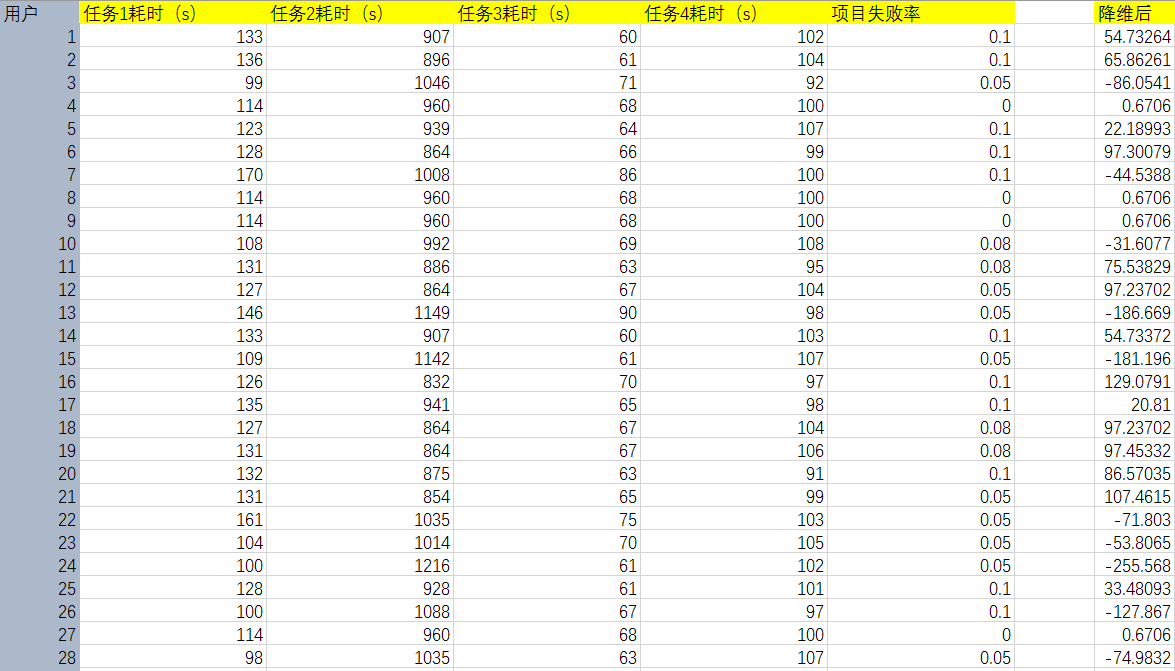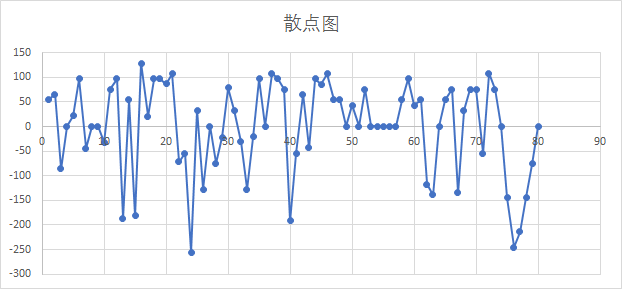1. 该App具有一定学习成本，新手导引需要改进，项目分布差值广泛
2. 该App在易用性上存在很大改进空间

### 转化矩阵P

1. 任务1与任务2在设计上存在冲突，若用户项目A上耗时时间短则在项目B上花费的时间就长。理由是：两者转换值为负相关。
2. 任务2，任务1是关键任务，需要在下一版本中着重设计。项目3，4影响不大，非关键任务，用户犯错率基本稳定。理由是：任务1的1s对应的数值是0.0535的数值变化而任务2中的1s对应的是-0.9984的数值变化。两者对于最后的分数的贡献是不一致的。

### 数据维度-碎石图

figure, plot(1:length(eigval),eigval,’ko-‘)
title(‘Scree Plot’)
xlabel(‘Eigenvalue Index – k’)
ylabel(‘Eigenvalue’)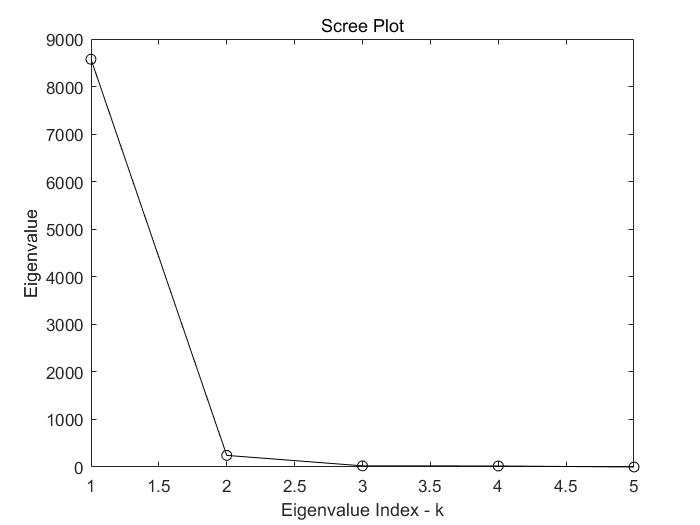## 后记

1. 项目之间的相关性（冲突还是互利）
2. 项目之间的重要层级和所影响的维度
3. 产品主要维度的构成

### 参考文献：

《Exploratory Data Analysis with MATLAB》 Wendy L. Martinez, Angel Martinez, Jeffrey Solka